Computer Aided Design - Fast Fourier Transform

# Computer Aided Design - Fast Fourier Transform - Notes | Study Signals and Systems - Electronics and Communication Engineering (ECE)

 1 Crore+ students have signed up on EduRev. Have you?

FIR filters can be useful in making computer-aided design of the filters. Let us take an example and see how it works. Given below is a figure of desired filter.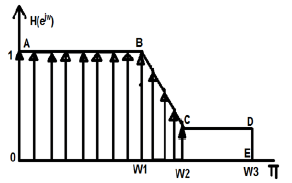While doing computer designing, we break the whole continuous graph figures into discrete values. Within certain limits, we break it into either 64, 256 or 512 (and so on) number of parts having discrete magnitudes.

In the above example, we have taken limits between -π to +π. We have divided it into 256 parts. The points can be represented as H(0), H(1),….up to H(256). Here, we apply IDFT algorithm and this will give us linear phase characteristics.

Sometimes, we may be interested in some particular order of filter. Let us say we want to realize the above given design through 9th order filter. So, we take filter values as h0, h1, h2….h9. Mathematically, it can be shown as below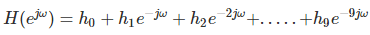Where there are large number of dislocations, we take maximum points.

For example, in the above figure, there is a sudden drop of slopping between the points B and C. So, we try to take more discrete values at this point, but there is a constant slope between point C and D. There we take less number of discrete values.

For designing the above filter, we go through minimization process as follows;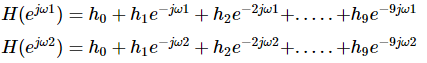Similarly,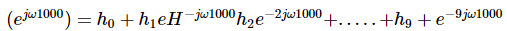Representing the above equation in matrix form, we have −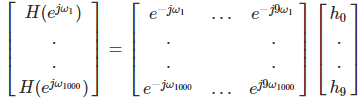Let us take the 1000×1 matrix as B, 1000×9 matrix as A and 9×1 matrix as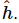So, for solving the above matrix, we will write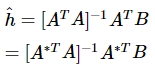where A* represents the complex conjugate of the matrix A.

The document Computer Aided Design - Fast Fourier Transform - Notes | Study Signals and Systems - Electronics and Communication Engineering (ECE) is a part of the Electronics and Communication Engineering (ECE) Course Signals and Systems.
All you need of Electronics and Communication Engineering (ECE) at this link: Electronics and Communication Engineering (ECE)

## Signals and Systems

32 videos|76 docs|63 tests
 Use Code STAYHOME200 and get INR 200 additional OFF

## Signals and Systems

32 videos|76 docs|63 tests

Track your progress, build streaks, highlight & save important lessons and more!

,

,

,

,

,

,

,

,

,

,

,

,

,

,

,

,

,

,

,

,

,

;